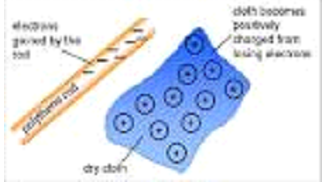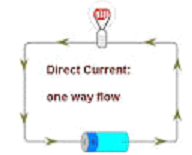# ELECTRICITY

## Electricity of Class 9

The fundamental principles to understand charges either positive or negative are coulomb’s law. If a charge particles moves then it results as current.

Electricity has an important role in modern society. In a span of more than 900 years, electricity convenient and widely used forms of energy in the world. One of the practical advantages of electricity as a form of energy is that it can readily transmitted over considerable distances with relatively small loss in energy.

This makes it possible to supply electricity from a central generating plant to any location. A type of energy fueled by the transfer of electrons from positive and negative points within a conductor. Electricity is widely used for providing power to buildings, electric devices, and even some automobiles.

A number of individuals are responsible for the development of electricity, but the most notable one is Benjamin Franklin and his flying kite experiment. Franklin was able to determine that lightning was a form of electrical discharge. Electricity are of two types as listed here under:

• Static Electricity
• Current Electricity

### STATIC ELECTRICITY

A Physical phenomena produced due to charges at rest is known as static electricity.Eg. When we rub a glass rod with silk the glass rod gets positive charge on it and equal and opposite charge (i.e. the -ve charge) gets developed on the silk. Similarly on a rubbing an ebonite rod with the fur of a cat the ebonite rod get negative charge on it and equal and opposite charge (i.e. the +ve harge) gets developed on the fur of a cat.

### CURRENT ELECTRICITY:

A Physical phenomena produced due to charges in motion is known as current electricity. e.g. electric current we use in our houses is current electricity.Now we will understand how these charges produced electricity.

### Potential difference

• The potential difference between the two conductors equals the work done in transferring the charging unit from one conductor to the other using a metal wire.
• V = W / q
• It is a scalar quantity. Its SI unit is volt (V).
• The potential difference between the two points is 1 volt if the work done on transferring 1 coulomb of charge from one point to another is 1 joule.

### Electrical Resistance

• There are always obstacles to the current flowing in the conductor, like a metal wire, which is called electrical resistance.
• According to Ohm's law, if the current I flow to a wire where the potential difference in the ends of the wire is V, then the resistance provided by the wire is.
• Resistance (R) = Potential difference in all wires (V) / Current flow in wire (l)

Q1. Define electric cell?

Ans. It is an electric device that converts chemical energy into electrical energy when it sends current in a circuit.

Q2. Name the constituents of a cell?

Ans. A cell constitutes of the following:

1. Two electrodes
2. An electrolyte in a vessel

Q3. What is a secondary cell?

Ans. Secondary cells or accumulators provide current as a result of a chemical reaction. The chemical reaction is reversible in these cells and can be recharged after use. One such cell is a Lead accumulator.

Q4. Define the term potential difference?

Ans. The potential difference between two conductors equals the work done in transferring a positive unit charge from one conductor to the other. It is a scalar quantity.

Q5. State Ohm’s law?

Ans. Ohm’s law states that if a current I flows through a wire when the potential difference across the ends of the wire is V, the resistance offered by the wire to the flow of current is the ratio of potential difference across it to the current flowing in it.

Q6. What do you mean by efficient use of energy?

Ans. It means reducing the cost and amount of energy used to provide various products and services.

To score More in your class 9 refer NCERT Solutions for Class 9. And If any students need to take the online test to check their concepts or undertstanding then they can visit Quiz for Electricity.